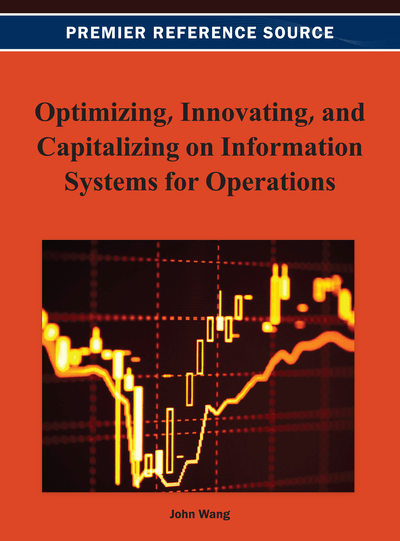# Bounded Primal Simplex Algorithm for Bounded Linear Programming with Fuzzy Cost Coefficients

DOI: 10.4018/978-1-4666-2925-7.ch003
OnDemand:
(Individual Chapters)
Available
\$29.50
No Current Special Offers

## Abstract

In most practical problems of linear programming problems with fuzzy cost coefficients, some or all variables are restricted to lie within lower and upper bounds. In this paper, the authors propose a new method for solving such problems called the bounded fuzzy primal simplex algorithm. Some researchers used the linear programming problem with fuzzy cost coefficients as an auxiliary problem for solving linear programming with fuzzy variables, but their method is not efficient when the decision variables are bounded variables in the auxiliary problem. In this paper the authors introduce an efficient approach to overcome this shortcoming. The bounded fuzzy primal simplex algorithm starts with a primal feasible basis and moves towards attaining primal optimality while maintaining primal feasibility throughout. This algorithm will be useful for sensitivity analysis using primal simplex tableaus.
Chapter Preview
Top

## Introduction

Recently various attempts have been made to study the solution of fuzzy mathematical programming problems, either from theoretical or computational point of view based on the fuzzy set theory (Zadeh, 1965). The concept of fuzzy mathematical programming on general level was first proposed by Tanaka, Okuda, and Asai (1974) in the framework of the fuzzy decision of Bellman and Zadeh (1970). The first formulation of Fuzzy Linear Programming (FLP) is proposed by Zimmermann (1978). Afterwards, many authors considered various types of the FLP problems and proposed several approaches for solving these problems. Chanas (1983) showed an application of parametric programming techniques in FLP and obtained the set of solutions maximizing the objective function, being analytically dependent on a parameter. Delgado, Verdegay, and Vila (1989) studied a general model for FLP problems which includes fuzziness both in the coefficients and in the accomplishment of the constraints. Buckley and Feuring (2000) considered the extreme case of fully fuzzified linear program with all the parameters and variables as fuzzy numbers. They turned the problem into a multi-objective FLP one. They showed that the fuzzy flexible programming could be used to explore the nondominated set to the multi-objective problem and proposed an evolutionary algorithm to solve the fuzzy flexible program. Jimenez, Cadenas, Verdegay, and Sanchez (2003) considered mathematical programming problems with fuzzy constraints. They obtained fuzzy solution by means of a parametric approach in conjunction with evolutionary techniques. Ganesan and Veeramani (2006) introduced a new approach for solving a kind of linear programming involving symmetric trapezoidal fuzzy numbers without converting them to the crisp linear programming problems. Nasseri and Mahdavi-Amiri (2009) based on this approach defined a new concept of duality of these problems. To deal with imprecise data, Wang and Liang (2009) introduced the notion of fuzziness and developed a procedure to provide finer evaluation results of Decision-Making Units (DMUs) with fuzzy observations based on the original context- dependent Data Envelopment Analysis (DEA) by using a ranking method based on the comparison of-cuts. Pahlavani (2010) presented a model to support the banking managerial decisions in the evaluation of investment plans, especially on rejecting inappropriate plans that can be done in short time (less than hour) and with minimal cost. Because there were some uncertainties in the evaluation process, its proposed model utilized fuzzy set theory to define the problem space in which an acceptance or rejection decision for a submitted investment plan had been made.

## Complete Chapter List

Search this Book:
Reset